## In-class worksheet 4

Jan 31, 2019

We will again be working with the ggplot2 package, so we need to load it:

``````library(ggplot2) # load ggplot2 library
theme_set(theme_bw(base_size = 12)) # set the default plot theme for the ggplot2 library``````

## 1. Bar plots

The `bacteria` data set contains data from tests of the presence of the bacterium H. influenzae in children with otitis media in the Northern Territory of Australia. We are interested in two columns of this data set: `presence` reports the presence (`y`) or absence (`n`) of the bacterium. `treatment` reports the treatment, which was `placebo`, `drug`, or `drug+` (drug plus high adherence).

``````# download the bacteria data set:
bacteria <- read.csv("http://wilkelab.org/classes/SDS348/data_sets/bacteria.csv")
head(bacteria)``````
``````##   presence ap hilo week  ID treatment
## 1        y  p   hi    0 X01   placebo
## 2        y  p   hi    2 X01   placebo
## 3        y  p   hi    4 X01   placebo
## 4        y  p   hi   11 X01   placebo
## 5        y  a   hi    0 X02     drug+
## 6        y  a   hi    2 X02     drug+``````

Using `geom_bar()`, make a bar plot that shows the absolute number of cases with or without the bacterium, stacked on top of each other, for each treatment.

``````ggplot(bacteria, aes(x = treatment, fill = presence)) +
geom_bar()``````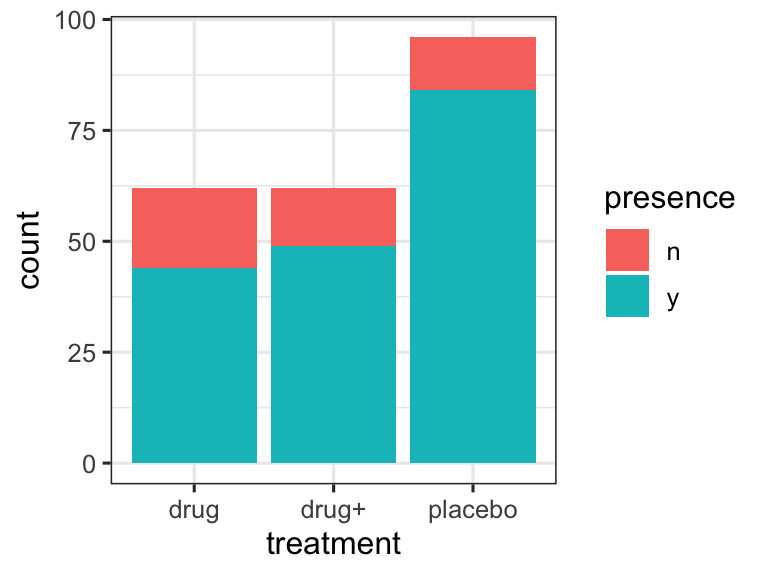Now modify the plot so that bars representing the absolute number of cases with or without the bacterium are shown side-by-side. Hint: This requires the argument `position='dodge'` in `geom_bar()`.

``````ggplot(bacteria, aes(x = treatment, fill = presence)) +
geom_bar(position='dodge')``````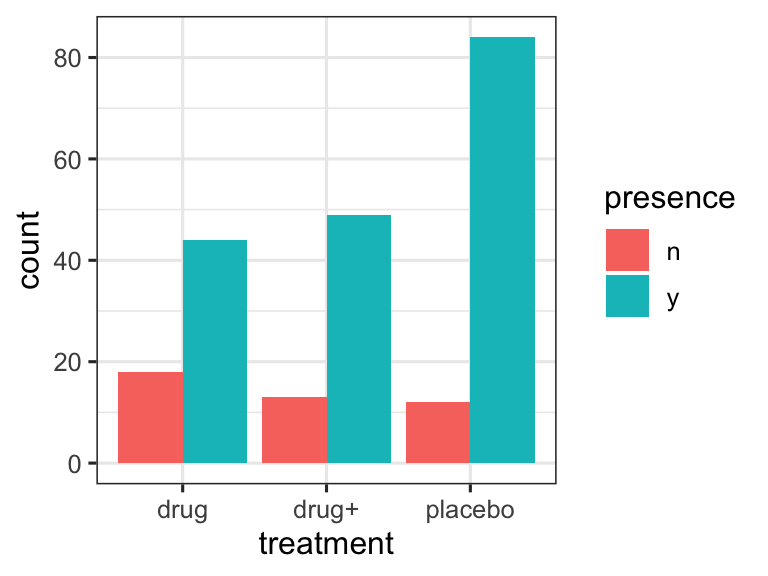Now modify the plot so that bars represent the relative number of cases with or without the bacterium. What is the appropriate `position` option in `geom_bar()` to achieve this effect?

``````ggplot(bacteria, aes(x = treatment, fill = presence)) +
geom_bar(position='fill')``````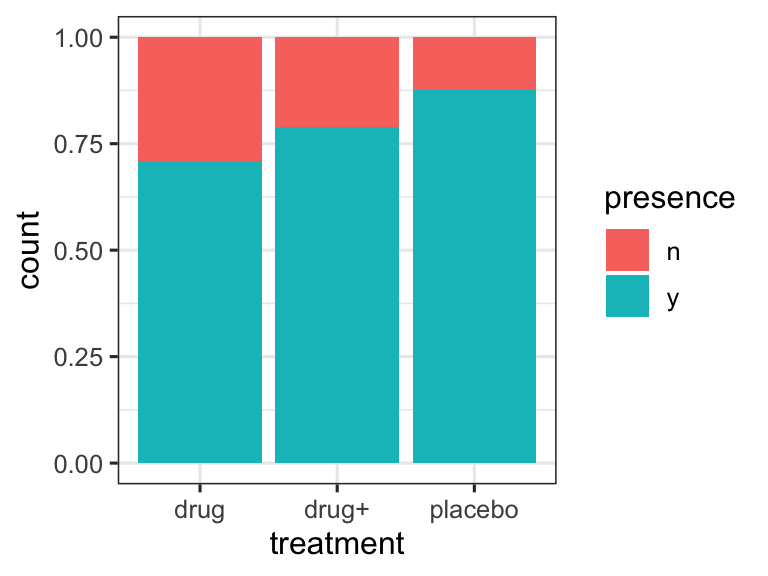## 2. Histograms and density plots

Make a histogram plot of sepal lengths in the `iris` data set, using the default histogram settings. Then make two more such plots, with different bin widths. Use `geom_histogram()`

``````# default settings
ggplot(iris, aes(x = Sepal.Length)) +
geom_histogram()``````
``## `stat_bin()` using `bins = 30`. Pick better value with `binwidth`.``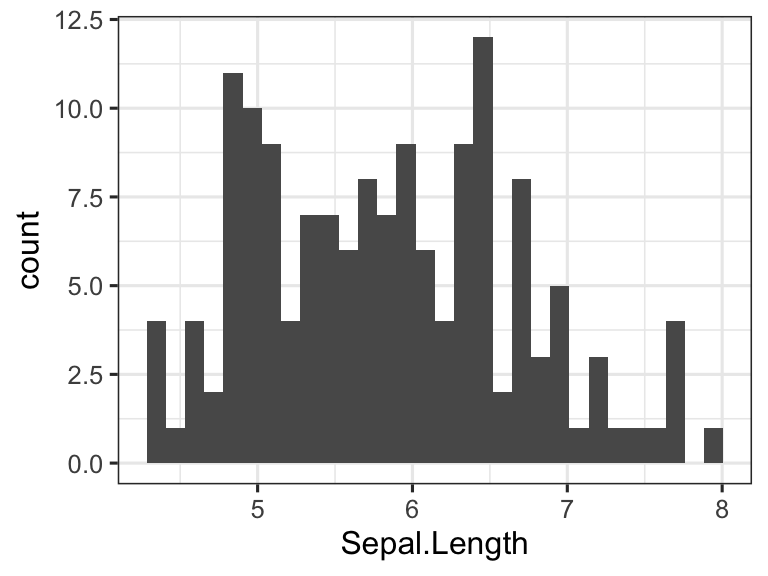``````# wider bins
ggplot(iris, aes(x = Sepal.Length)) +
geom_histogram(binwidth = 0.2)``````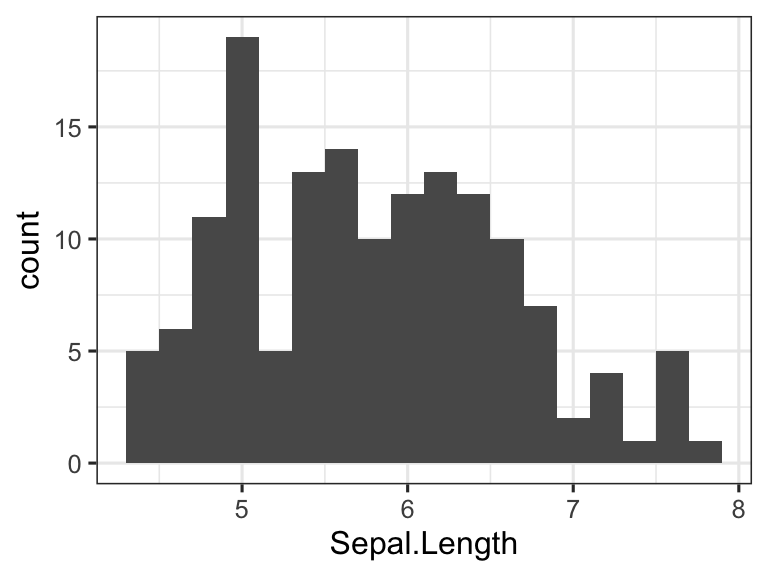``````# even wider bins
ggplot(iris, aes(x = Sepal.Length)) +
geom_histogram(binwidth = 0.4)``````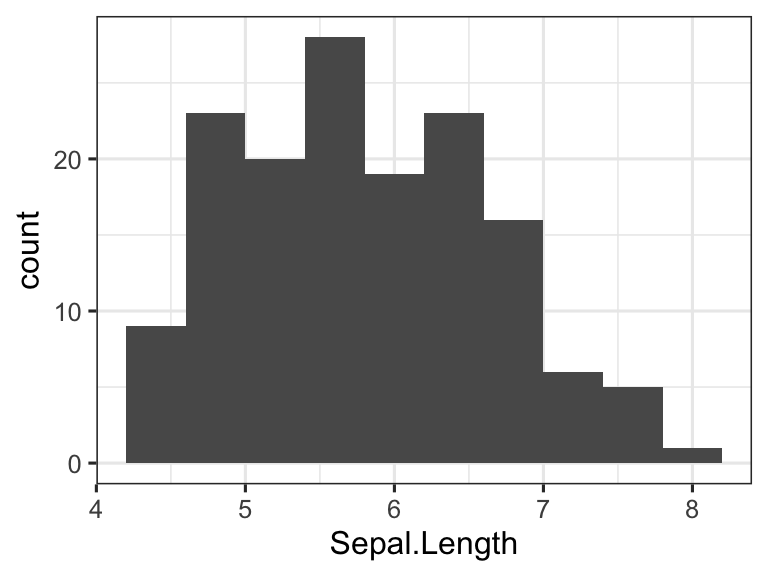Instead of `geom_histogram()`, now use `geom_density()` and fill the area under the curves by species identity.

``````ggplot(iris, aes(x = Sepal.Length, fill = Species)) +
geom_density()``````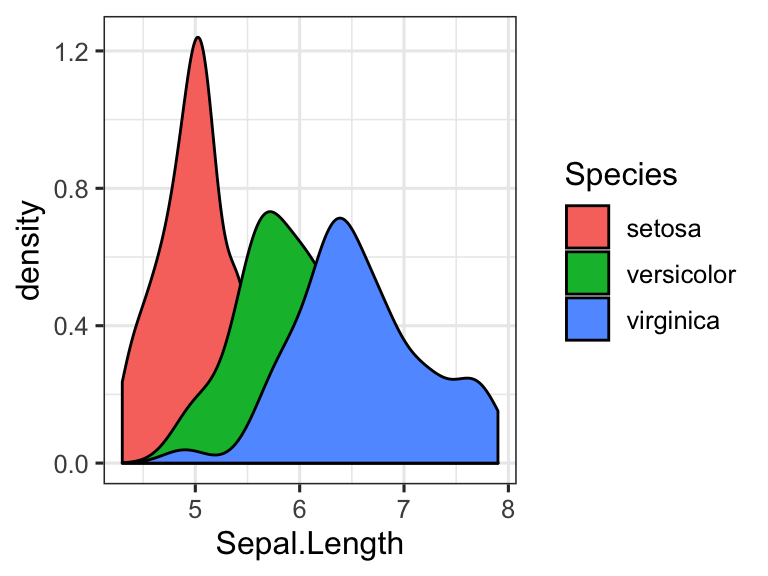Now make the areas under the curve partially transparent, so the overlap of the various distributions becomes clearly visible.

``````ggplot(iris, aes(x = Sepal.Length, fill = Species)) +
geom_density(alpha = 0.7)``````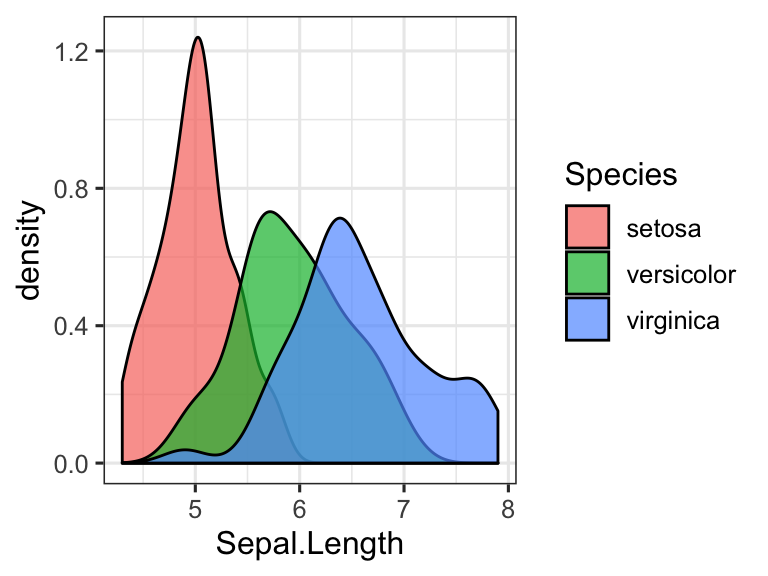## 3. Scales

The `movies` data set provided in the package ggplot2movies containes data from the internet movie database (IMDB) about 28819 different movies. It contains information such as the length of the movie, the year the movie was released, number of votes the movie has received on the IMDB, and so on. To use the data set, you first need to load it in:

``````library(ggplot2movies)
head(movies)``````
``````## # A tibble: 6 x 24
##   title  year length budget rating votes    r1    r2    r3    r4    r5
##   <chr> <int>  <int>  <int>  <dbl> <int> <dbl> <dbl> <dbl> <dbl> <dbl>
## 1 \$      1971    121     NA    6.4   348   4.5   4.5   4.5   4.5  14.5
## 2 \$100…  1939     71     NA    6      20   0    14.5   4.5  24.5  14.5
## 3 \$21 …  1941      7     NA    8.2     5   0     0     0     0     0
## 4 \$40,…  1996     70     NA    8.2     6  14.5   0     0     0     0
## 5 \$50,…  1975     71     NA    3.4    17  24.5   4.5   0    14.5  14.5
## 6 \$pent  2000     91     NA    4.3    45   4.5   4.5   4.5  14.5  14.5
## # … with 13 more variables: r6 <dbl>, r7 <dbl>, r8 <dbl>, r9 <dbl>,
## #   r10 <dbl>, mpaa <chr>, Action <int>, Animation <int>, Comedy <int>,
## #   Drama <int>, Documentary <int>, Romance <int>, Short <int>``````

Now, using this data set, make a scatter plot of the number of votes (`votes`) vs. the length of the movie (`length`). Use a log scale for both the x and the y axis.

``````ggplot(movies, aes(y = votes, x = length)) +
geom_point() +
scale_x_log10() +
scale_y_log10() ``````